# Erroneous results when dealing with small values

Hello Everyone,

When I am trying to add / aggregate small double values, I am getting discrepancies. Though this might be because how double datatype is stored.
However,When I adding these values using groupby or math formula node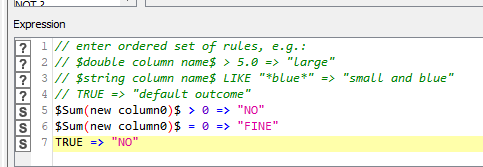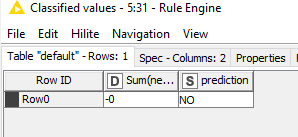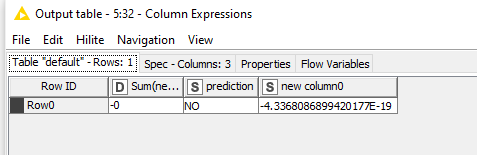Instead of 0 I am getting vey small value 10^-19 which in normal cases is alright.

However, we are using a rule engine to flag values which are greater than 0 and using those values we are dividing it with another number.

For example here 3/(-4.3368086899420177E-19)

But due to this we are getting very large numbers and getting wrong results.

Is there any other datatype we need to use in these kinds of situations ?
or is there any other way to go in this scenario ?

This seems to me to be a limitation of the double-precision floating point number format. Trying to represent that many significant digits after a decimal point leads to a loss of information. Not every decimal number can be expressed exactly as a floating point number

I’m not sure that anything can be done about it besides maybe adding rounding step.

4 Likes

Hi @berserkersap , this indeed seems like it’s cause due to the limitation of the floating point precision as @elsamuel mentioned.

Depending on what you need the value for and what you are validating, to help with the precision, you can always multiply by something like 10000 or even 100000, or even 1000000. Most certainly you want to do the multiplication before the division.

Your rules might still be usable as is. The worst case would be you can just also multiply your rules by whatever you multiplied in the calculation.

2 Likes

Hello @bruno29a , I was also considering that. However, we have values ranging from 10^8 to 10^-7 with a accuracy of 7 decimals.
The sum of those can go till 10^18
If I want to multiply the numbers, I need to multiply with 10^5 at the very minimum. I need to check if there is no loss of digits if the value goes to 10^23 (18+5).

When I tried this in table creator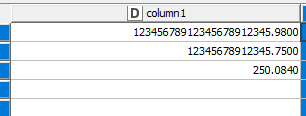The executed values are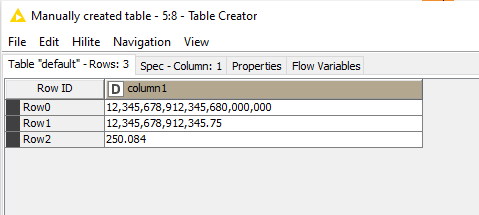I can see KNIME rounded of the value in row 0 . So it seems it will only take 16 digits and rest of them Zeroes.

Sum of the numbers

Sum of the numbers by calculator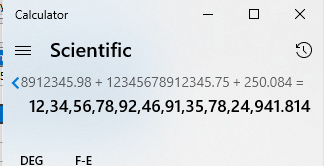Well though I need to again check if this is feasible.
Now I have a doubt, how do people work with this, like when they need more accurate numbers (may be in medical, research, accounting and so on)

Are you able to take the logarithm of your data and use that? That is what most do in a situation where the data has a high dynamic range (e.g. decibels). The other alternative would be software packages such as Mathematica that have capabilities for high precision calculations.

4 Likes

Hello @DiaAzul ,
Thank you for the answer. I will try using logarithm but probably might not use it in the end.
I thought softwares like Mathematica are used for differentiation, integration that sort of mathematical functions . Will that also be suitable for this requirement ?

@berserkersap

Mathematica is a symbolic and numerical computation programming language with an extensive library of capabilities. It introduced the notebook approach to programming (copied by Jupyter) and is a functional programming language, like Julia. The library of available functions is extensive and includes a wide range of statistics, machine learning and visualisation components amongst many others. The downside is that the language is not very readable, which makes development and debugging painful. It is also expensive (relative to other options such as R, Python and KNIME).

What you have described so far are the symptoms of your problem, not what you are trying to achieve. That is, the analysis you are doing is generating very large and small numbers, which is the symptom; the algorithm (what you are doing) is the cause. If you are able to share more about what it is you are trying to do and how you are going about doing it then the forum might have better suggestions. I appreciate this may not be possible if there are issues of confidentiality.

Hello @DiaAzul ,
When you speak of Mathematica, it is Wolfram Mathematica right ?
It is true that I cannot tell the requirements due to confidentiality.
But I can just say we have tabular data (few text columns, few columns with numeric data) , joins, group bys, multiplication, division, rule engines and we need accurate results.
I know this is pretty vague, sorry about that

@berserkersap , thanks for the extra information, that helps a little.

What you could do is store the numbers in KNIME as a string representation. This would preserve their accuracy whilst you performed you data joins and manipulations. When you reach the point that you need to perform a calculation you can pass the data (numbers as strings) into a Python node.

Within the Python node you can use the decimal library functions to perform exact mathematical calculations at the required precision. The library allows you to set the precision of the calculation to match your needs. You can then output from the Python node as either text representation of the number (to preserve precision) or as a Java decimal number (in which case rounding may occur).

The documentation for Python decimal is here : decimal — Decimal fixed point and floating point arithmetic — Python 3.10.6 documentation

If you use the Python (labs) node you can batch the processing if you have a lot of data. I am sure that you could figure out the best way to build this into your workflow.

Best regards.

2 Likes

Thanks @DiaAzul ,
Sounds like a plan. I will try and let you know.
However, when I tried this library in a online compiler, it is still not completely accurate.

@berserkersap
You need to enter the values as strings. The example you have given has the numbers converted to Python floats before they are converted to Decimal. You are losing precision at this stage. If you enter the values as strings it should compute correctly.

Thank you very much @DiaAzul , this might work.I never thought even my input was first being taken as floating point valueHonestly, this is my first time working with python with KNIME and need to check the environment and all but I can take care from here

1 Like

Hello @DiaAzul ,
Sorry to bother you again but do you know how to use group by and pivot in python node while maintaining the decimal data type (the accuracy)?

@berserkersap

I’ve created an example workflow on KNIME hub showing the basic principles.
Decimal Pivot Test Workflow

The workflow creates dummy data consisting of one column of fruit, one column of furniture and one column of values (random decimal numbers in KNIME converted to strings). The table has 3,000 rows.

Fruit Furniture StringVals
Orange Bed 0.9829986280644677
Pear Drawers 0.11667831727663647
Apple Drawers 0.10520710604664196
Banana Bed 0.8880643569601723
Orange Bed 0.061570716347171595
Grapefruit Sofa 0.156195989186808

The first node uses Pandas groupby function to group the data into the 25 fruit-furniture combinations and sums the values.

The basic principles are:

1. Convert the string values to decimals using the apply() method on the DataFrame.
2. Drop the column with string values.
3. Group the data.
4. Convert the decimal values back to strings.
5. Drop the decimal values from the DataFrame.
6. (Optional). Pandas takes the grouping keys and uses them to index the rows in the resultant DataFrame. This DataFrame can be exported directly to KNIME, however, the row indexes will become RowIDs. It may be more convenient to export the index to columns and reset the index in the DataFrame. This will match the format of output produced by the KNIME groupby node.

The Python script for GroupBy is below.

``````import knime_io as knio
import pandas as pd
from decimal import Decimal

input_table = knio.input_tables.to_pandas()

# Convert values in string column to decimal
input_table["DecVals"] = input_table["StringVals"].apply(lambda x: Decimal(x))
# Drop the string column (we don't need it in the grouping.
pre_group = input_table.drop(["StringVals"], axis=1)
# Group the data on two keys Fruit and Furniture. Sum the values.
grouped_data = pre_group.groupby(["Fruit", "Furniture"]).sum()

# Convert the decimal back to a string value and drop the decimal column.
grouped_data["StringVals"] = grouped_data["DecVals"].apply(lambda x: str(x))
string_data = grouped_data.drop(["DecVals"], axis=1)

# The grouping keys become row index in python -> Will also be RowID.
# Unless we extract index to columns and reset the index.
output_data = string_data.reset_index(level=['Fruit', 'Furniture'])

knio.output_tables = knio.write_table(output_data)
``````

The second node provides an example of pivoting the data. This follows a very similar pattern to the groupby example, with the exception that converting the decimal values back to strings is more difficult. In the groupby example there is only one value column. Therefore, when we apply the str(x) function it is applied to the value in that row, which is unique. However, if there is more than one column then pandas passes the entire row to the str() function which converts the values to decimal and concatenates the string. Therefore, when there is more than one column of data we need to first take each row in the data frame (iterate across the y axis) and then iterate across the values in that row (iterate across the x axis). This then generates a new table (`string_data`) with decimal values converted to strings.

The pivot_table example is below.

``````import knime_io as knio
import pandas as pd
from decimal import Decimal

input_table = knio.input_tables.to_pandas()

# Convert values in string column to decimal
input_table["DecVals"] = input_table["StringVals"].apply(lambda x: Decimal(x))
# Drop the string column (we don't need it in the grouping.
pre_pivot = input_table.drop(["StringVals"], axis=1)

# Pivot the data rows for Furniture and columns for Fruit.
grouped_data = pre_pivot.pivot_table(
values="DecVals",
index=["Furniture"],
columns=["Fruit"],
aggfunc=sum
)

# Convert the decimal back to a string value.
# Note: The first lambda function take each column (y) in turn and passes
# it to the second lambda function that works down the row and converts each
# decimal value to a string.
string_data = grouped_data.apply(lambda y: y.apply(lambda x: str(x)))

# The grouping keys become row index in python -> Will also be RowID.
# Unless we extract index to columns and reset the index.
output_data = string_data.reset_index(level=['Furniture'])

knio.output_tables = knio.write_table(output_data)
``````

I hope that helps.
DiaAzul.

4 Likes

Thank you very very much @DiaAzul !, this is really helpful.

I wasn’t that proficient in python1 Like

Hi

From what I remember a long time ago from my numerical computation class:

• Can you use integers, perhaps by scaling all your numbers. Probably this will not work but it is worth mentioning

• Sort your data by absolute magnitude, with numbers of smallest magnitude first. Then perform your calculation (which I didn’t read). The idea behind this is that you can sum up the numbers with lower magnitudes first, and then proceed with numbers of larger magnitudes. You should be able to maximize the actual precision saved.

• Write your own node using Java and use classes like java.math which will allow you arbitrary precision integer or decimal arithmetic. You can use a separate variable for the exponent. FYI, java.math is not the only available class, see also Java floating point high precision library - Stack Overflow

1 Like

Are you able to take the logarithm of your data and use that?

This topic was automatically closed 7 days after the last reply. New replies are no longer allowed.